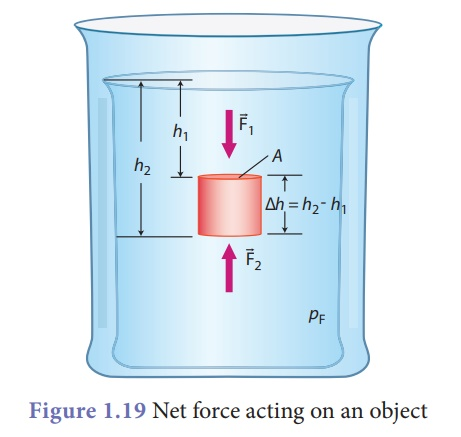Home | | Science 9th std | Buoyancy

# Buoyancy

Most buoyant objects are those with a relatively high volume and low density. If the object weighs less than the amount of water it has displaced (density is less), buoyant force will be more and it will float (such object is known as positively buoyant). But if the object weighs more than the amount of water it has displaced (density is more), buoyant force is less and the object will sink (such object is known as negatively buoyant).

Buoyancy

We already saw that a body experiences an upward force due to the fluid surrounding, when it is partially or fully immersed in to it. We also saw that pressure is more at the bottom and less at the top of the liquid. This pressure difference causes a force on the object and pushes it upward. This force is called buoyant force and the phenomenon is called buoyancy (Fig.1.17).Most buoyant objects are those with a relatively high volume and low density. If the object weighs less than the amount of water it has displaced (density is less), buoyant force will be more and it will float (such object is known as positively buoyant). But if the object weighs more than the amount of water it has displaced (density is more), buoyant force is less and the object will sink (such object is known as negatively buoyant).

### Cartesian diver

Cartesian diver is an experiment that demonstrates the principle of buoyancy. It is a pen cap with clay. The Cartesian diver contains just enough liquid that it barely floats in a bath of the liquid; its remaining volume is filled with air. When pressing the bath, the additional water enters the diver, thus increasing the average density of the diver, and thus it sinks.Examples 1.9

Six objects (A-F) are in a liquid, as shown below. None of them are moving. Arrange them in order of density, from lowest to highest.Solution:

The more of an object’s volume is above the water surface, the less dense it is. Object B must therefore be the least dense, followed by D, A, and F. Object E is next, because it is neutrally buoyant and equal in density to the liquid. Object C is negatively buoyant because it is denser than the fluid.

Therefore the order of density from lowest to highest is B,D,A,F, E,C.

## Mathematical representationof Buoyant force

For an object submerged in a fluid, there is a net force on the object, because the pressure at the top and bottom of it are different.We know that pressure, P = F/A

F = P A

Fbuoyancy = F2 – F1 = P2A2 – P1A1

Since area is same, A2 = A1 = A

Therefore,

Fbuoyancy = P2A – P1A

= A(P2 – P1)

Since, P = hρg,

Fbuoyancy = A(ρgh2 – ρgh1)

Aρg(h2 – h1) = ρgA(h2 – h1)

Fbuoyancy = ρg(AΔh) = (ρfluid) g (Vdisplaced)

### Example 1.10

A golden crown has been placed in a tub of water. The volume of water displaced is measured to be 1.50 liters. The density of water is 1000 kg m–3, or 1.000 kg L–1. What is the buoyant force acting on the crown?

### Solution:

The buoyant force is, Fb = ρgV

First, we ensure that the units used for volume are the same.

If 1 m3 = 1000 L, then 1.50 L = 0.00150 m3.

Fb = (1000 kg m–3)(9.80 m s-2)(0.00150 m3)

= 14.7 kg m s–2 = 14.7 N

The buoyant force acting on the golden crown is 14.7 N.

Study Material, Lecturing Notes, Assignment, Reference, Wiki description explanation, brief detail
9th Science : Fluids : Buoyancy |